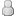• 1 Votes - 5 Average
• 1
• 2
• 3
• 4
• 5
01-18-2014, 09:27 AMPost: #39
 naoanMemberPosts: 71 Joined: Aug 2013 Reputation: 0
sure

Code:
[General]
FirstRun = False
RunCount = 586
Enable Logging = True
AutoRun = True
Browse = False
CurrentDirectory =
ReportingHost = default
AutoSaveSymbolMap = False
TopMost = False
WindowX = 369
WindowY = 40
WindowWidth = 968
WindowHeight = 594
PauseOnLostFocus = False
Language = en_US
EnableCheats = True
ScreenshotsAsPNG = True
StateSlot = 1
RewindFlipFrequency = 0
GridView1 = True
GridView2 = True
GridView3 = False
CheckForNewVersion = False
[Recent]
MaxRecent = 30
[PinnedPaths]
[CPU]
Jit = True
AtomicAudioLocks = False
FastMemoryAccess = True
CPUSpeed = 333
[Graphics]
ShowFPSCounter = 0
RenderingMode = 1
SoftwareRendering = False
HardwareTransform = True
SoftwareSkinning = True
TextureFiltering = 3
InternalResolution = 4
FrameSkip = 0
FrameRate = 0
FrameSkipUnthrottle = False
ForceMaxEmulatedFPS = 60
AnisotropyLevel = 4
VertexCache = True
FullScreen = True
PartialStretch = False
StretchToDisplay = False
TrueColor = True
MipMap = True
TexScalingLevel = 4
TexScalingType = 0
TexDeposterize = False
VSyncInterval = True
DisableStencilTest = False
AlwaysDepthWrite = False
TimerHack = True
LowQualitySplineBezier = False
TextureBackoffCache = True
TextureSecondaryCache = True
SmallDisplay = False
[Sound]
Enable = True
VolumeBGM = 7
VolumeSFX = 7
LowLatency = False
[Control]
HapticFeedback = True
ShowTouchControls = False
ShowTouchCross = True
ShowTouchCircle = True
ShowTouchSquare = True
ShowTouchTriangle = True
ShowTouchStart = True
ShowTouchSelect = True
ShowTouchLTrigger = True
ShowTouchRTrigger = True
ShowAnalogStick = True
ShowTouchUnthrottle = True
TouchButtonStyle = 1
TouchButtonOpacity = 65
ActionButtonScale = 1.150000
ActionButtonSpacing2 = 1.000000
ActionButtonCenterX = 0.875000
ActionButtonCenterY = 0.779412
StartKeyX = 0.637500
StartKeyY = 0.873162
StartKeyScale = 1.150000
SelectKeyX = 0.500000
SelectKeyY = 0.873162
SelectKeyScale = 1.150000
UnthrottleKeyX = 0.361458
UnthrottleKeyY = 0.873162
UnthrottleKeyScale = 1.150000
LKeyX = 0.083333
LKeyY = 0.084559
LKeyScale = 1.150000
RKeyX = 0.928125
RKeyY = 0.084559
RKeyScale = 1.150000
AnalogStickX = 0.148958
AnalogStickY = 0.830882
AnalogStickScale = 1.150000
IgnoreWindowsKey = False
[Network]
EnableWlan = False
[SystemParam]
PSPModel = 1
PSPFirmwareVersion = 150
NickName = PPSSPP
Language = 1
TimeFormat = 2
DateFormat = 1
TimeZone = 0
DayLightSavings = False
ButtonPreference = 0
LockParentalLevel = 0
WlanPowerSave = False
EncryptSave = True
BypassOSKWithKeyboard = False
[Debugger]
DisasmWindowX = 140
DisasmWindowY = 45
DisasmWindowW = 773
DisasmWindowH = 622
GEWindowX = 200
GEWindowY = 86
GEWindowW = 768
GEWindowH = 688
ConsoleWindowX = -1
ConsoleWindowY = -1
FontWidth = 8
FontHeight = 12
DisplayStatusBar = True
ShowBottomTabTitles = True
FuncHashMap = False
[SpeedHacks]
PrescaleUV = False
DisableAlphaTest = False